HOME plateWON | World!OfNumbers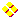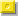Palindromic Pronic Numbersof the form n(n+1)n(n+2)n(n+x)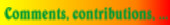Introduction

Palindromic numbers are numbers which read the same from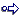left to right (forwards) as from the right to left (backwards)Here are a few random examples : 535, 3773, 246191642

Palindromic Pronic Numbers are defined and calculated by this extraordinary intricate and excruciatingly complex formula.
So, this line is for experts onlybase x ( base + n )
In case of n = 1 we speak about our 'pronic' numbers !
Palindromic Pronic Numbers can only begin (and end!) with the digits 2 and 6
and if it terminates in 6, it terminates in 06 or 56.
Every pronic number is the double of a triangular. A quick look at both formulae reveals this fact :
n(n+1)/2 versus n(n+1).

To know more about the case whereby n = 2 visit this page named Palindromic Quasipronic Numbers.

Palindromic Pronic NumbersSo far I compiled more than 60 palindromic pronic numbers.
Here is the largest one which was submitted to me by Warut Roonguthai
from Bangkok (Thailand) on [ August 31, 1997 ].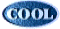This (palindromic!) basenumber 255.455.445.544.554.455.445.544.554.552 has 30 digits yielding the following extraordinary palindromic pronic record number (ppn) 65.257.484.658.366.826.781.688.069.846.664.896.088.618.762.866.385.648.475.256 with a length of (hold your breath) 59 digits.

Warut Roonguthai (website) found this record number not by letting his UBASIC program perform an exhaustive search but by 'computer-assisted construction'.
He probably saw the following pattern emerging after finding these two ppn's :

 2554554552
 25545544554552
Could it be that he noticed that the second basenumber equals the first one with a core number 5445 added in between ?
25545|54552
2554554|4554552
Excited by this initial discovery maybe he tried to find an expansion by setting up a grow-pattern.
Perhaps he succeeded after realising he had to use two alternate core numbers 5445 and 4554 !
That insight paves the way to construct ppn's upto the record one :
255455445|544554552
25545544554|45544554552
2554554455445|5445544554552
255455445544554|455445544554552
It's a pity that this remarkable expansion is finite.
Note that the last basenumber has 30 digits and leads sofar to the largest constructed palindromic number of my whole website nl. 59 digits. Quite an achievement !

A similar procedure applied on the non-palindromic basenumber 31 and using core numbers 4455 and 5544 gave birth to more palindromic pronic numbers :
 255455445447
255455|445447
25545544|55445447
2554554455|4455445447
255455445544|554455445447
25545544554455|44554455445447

Warut emailed me after reading all these assumptions and wrote that the way he recognized the patterns of the two ppn series is quite different from what I guessed above ! Actually he sees them as follows :
255 (4554)n 552, for n = 1 to 6
255 (4554)n 45447, for n = 1 to 5
Simplicity is the hall-mark of truth.
|Astonishing!|

Curious Pairs of Consecutives

16 & 17

Admire for a moment this extraordinary manyfold construction with Two Consecutive Integers 16 and 17.

16 x 17 = 272   [ or 16 + 162 ]

161 + 171 = 33
162 + 172 = 545
163 + 173 = 9009

171161 = 1
172162 = 33

and this bellying construction (finite, alas) where 16 and 17 embrace the numbers 70 is quite phenomenal !

162 + 172 = 545
17062 + 17072 = 5824285
1707062 + 1707072 = 58281418285
170707062 + 170707072 = 582818040818285

and that is not all because when we add more consecutives to the expression we see the following :

163 + 173 + 183 = 14841 (#3)
163 + 173 + ... + 253 + 263 = 108801 (#11)
163 + 173 + ... + 4073 + 4083 = 6961551696 (#393)
or summing the #311 squares from 16 up to 326
162 + 172 + ... + 3252 + 3262 = 11600611
See Powered consecutives summing to palindromes for the database.

Changing the cube to squares in the above expression allows us to stuff the three consecutive numbers with 73

17362 + 17372 + 17382 = 9051509
1737362 + 1737372 + 1737382 = 90553635509
The three consecutive numbers 16, 17 and 18 generate primes when applied to the formula n2 + n + 5.
Another such triplet is {191,192,193}, can you find more ?
162 + 16 + 5 = 277 is also a prime with multiplicative persistence of value 4 - see A034051.
172 + 17 + 5 = 311
182 + 18+ 5 = 347
Last one in the queue are these two polynomials whereby 16 and 17 embrace the number  87
18762 + 18771 = 3521253
1878762 + 1878771 = 35297579253

All above operations yield a palindromic result!

Note that 9009 can be expressed as the product of four consecutives i.e. the sequence (n) x (n+2) x (n+4) x (n+6)
or 9009 = 7 x 9 x 11 x 13

A remarkable similar repetitive infinite nonpalindromic pattern can be made with 16 and 17 in the following manner :

16 + 17 = 33
1706 + 1707 = 3413
170706 + 170707 = 341413
17070706 + 17070707 = 34141413
Chen Shuwen (email) of the People's Republic of China made a profound search for solutions of the Diophantine System.
In his website about Equal Sums of Like Powers I came across the following :
( k = 1,2 )
[ 0, 16, 17 ] = [ 1, 12, 20 ] = [ 2, 10, 21 ] = [ 5, 6, 22 ]
meaning :
33 = 0 + 16 + 17 = 1 + 12 + 20 = 2 + 10 + 21 = 5 + 6 + 22
545 = 02 + 162 + 172 = 12 + 122 + 202 = 22 + 102 + 212 = 52 + 62 + 222

The equation n2 + n + 17 is known to produce 16 primes for consecutive values of n from 0 to 15.
The integer sequence whereby %N n2 + n + 17 is composite. (Sloane's A007636) begins as follows :
%S 16,17,20,25,32,33,34,36,39,41,43,48,50,51,...

Making operations with the concatenation of our two consecutive numbers 16 and 17
gives rise to more palindromic outcome :

17161617 = 99
1716 + 1617 = 3333
1716 x 1617 = 2774772
GCD ( 1716 , 1617 ) = 33
1617 = the 33rd Pentagonal Number !
Note 1617 is also 7 times the 21st triangular number (7 x 231)
and 231 is the 16th partition number.
or play around with their reversals :
16 + 61 = 77
17 + 71 = 88
1617 + 7161 = 8778
1716 + 6171 = 7887
71176116 = 1001
17171616 = 101

1661 + 1771 = 3432 = 2 x 1716 !!!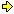Note that 1716 = 11 * 12 * 13 the product of three consecutive integers.
A small discovery made using Pi-Search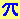The string 1771 was first found at position 8448 counting from the first digit after the decimal point.
The 3. is not counted.

Let me tell you a story about, by now, two wellknown consecutive numbers :

The smallest number one must multiply with 16 to arrive at a palindrome is 17.
16 x 17 = 272
The smallest number one must multiply with 17 to arrive at a palindrome is 16.
17 x 16 = 272

Let me retell the above story but now with the 'concatenated' numbers 16 and 17 :

The smallest number one must multiply with 1617 to arrive at a palindrome is 123.
1617 x 123 = 198891
The smallest number one must multiply with 1716 to arrive at a palindrome is 147.
1716 x 147 = 252252
Note that when we multiply these two multipliers together we find again a palindrome !
123 x 147 = 18081

On [ September 1st, 1997 ] Bill Taylor (email) posted the following puzzle in sci.math involving the numbers 16 and 17 :
Partition the integers 1 to 23 into three sets, such that for no set are there three different numbers with two adding to the third.
There are three solutions... (23 was the largest number for which this could be done.)

```*****************
1   2   4   8   11   16   22
3   5   6   7   19   21   23
9   10   12   13   14   15   17   18   20
*****************
1   2   4   8   11   17   22
3   5   6   7   19   21   23
9   10   12   13   14   15   16   18   20
*****************
1   2   4   8   11   22
3   5   6   7   19   21   23
9   10   12   13   14   15   16   17   18   20
*****************
```
It's intriguing to note that most of the integers are fixed,
with just 16 & 17 movable. (Bill doubts there's any real significance in this though.)

While skimming through the integer sequences in Sloane's table I came across entry number A006488.
The description is as follows : n! (n factorial) has a square number of digits.
The term 1617 pops up on the second line.
I asked Warut Roonguthai to calculate this square number for me.
He responded concise but to the point :
Since n! is asymptotically equal to (n/e)^n*sqrt(2*pi*n) (Stirling's formula),
we can find that log(1617!) is about 4.488,2.
So 1617! has 4489 = 672 digits.
All along the answer was hidden in the proper digits of the term itself !

The least integer such that the decimal part of its square root starts with
the ninedigits number 123456789 is, lo and behold [ Source: Solution to Problem #14 ]
1661201661617^(1/2) = 1288876.12345678900003617...
After careful observation we noticed the following facts :
1. the number begins with 16 and ends with 17.
1661201661617
2. we find twice the occurence of 1661.
1661201661617
3. and once the occurence of 1617.
1661201661617
4. the inverse reversal 6116 also occurs in a separated way.
1661201661617

Let me quote a paragraph from Theoni Pappas' Book "The Joy of Mathematics", Wide World Publishing/Tetra,
Edition 1998, page 3, from the very first chapter 'The evolution of Base Ten'.

"... and scientists were reticent to employ the base 10 system
because it had no simple way to denote fractions. But it became
popular when merchants adopted it, since it proved so
invaluable in their work and record keeping. Later, decimal
fractions made their appearance in the 16th century, and the
decimal point was introduced in 1617 by John Napier."

Here is a prime starting with 1716 having the interesting property
that it will remain prime when any of its digits is deleted.

1716336911

[ Source: Prime Curios! 1716336911 ]

A second interesting equation I found is this one.
Note the presence of '17' and '61' in the prime result.

17171616 = 808793517812627212561

[ Source: Prime Curios! 808793517812627212561 ]

We knew already that 16 + 17 = 33
Did you know, a nice pattern exists, taken from WONplate 152, i.e.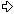Near_tautonymic numbers of the form (T)_(T + 1) as SQUARES
(4n)5(3n)2(8n)8_(4n)5(3n)2(8n)9 = (6n)7(3n)2(6n)72
Among the first 33333 values of n only 0, 16, 17, 33, 2738, 3096 yield PRIMES for the
right part of the equation ! Note the succession of the same three values 16, 17 and 33.
n = 16
prime ^ 2 = 666666666666666673333333333333333266666666666666667 ^ 2 =
444444444444444453333333333333333288888888888888888_444444444444444453333333333333333288888888888888889
n = 17
prime ^ 2 = 666666666666666667333333333333333332666666666666666667 ^ 2 =
444444444444444445333333333333333332888888888888888888_444444444444444445333333333333333332888888888888888889

1617 occurs many times as displacement to powers of ten
so that this number forms a (probable) prime nearest to those axes.

10293 + 1617 is the smallest prime of length 294.
10388 + 1617 is the smallest prime of length 389.
101276 + 1617 is the smallest prime of length 1277.
101651 + 1617 is the smallest prime of length 1652.
102416 + 1617 is the smallest prime of length 2417.
106689 + 1617 is the smallest prime of length 6690.
109109 + 1617 is the smallest prime of length 9110.
1018448 + 1617 is the smallest prime of length 18449.
1025344 + 1617 is the smallest prime of length 25345.
1028725 + 1617 is the smallest prime of length 28725.
1041905 + 1617 is the smallest prime of length 41906.

A prime gap of  1716 occurs twice
between the following nearest primes around powers of ten :
Between 101653927 and 101653 + 789 (difference is 1716)
Between 101857137 and 101857 + 1579 (difference is 1716)

162 + 172 = 545

Now Jean-Claude Rosa found an impressive extension of the expression of the square of two consecutives
[ Source : sumsquare.htm ]

16508893703300162 + 16508893703300172 = 5450871426137276727316241780545

Note that 16 and 17 appear also in the decimal expansion of the palindrome !

From wonplate 197 there is this nice FEP (First Encountered Prime) duo :
We have not only 16 + 1 = 17 but also 1945 + 1 = 1946 !
[1_16] = prime!
[1_17] = prime!
One needs to add at least 16 ones to 1945 to arrive at a prime.
One needs to add at least 17 ones to 1946 to arrive at a prime.
Can you find the next couple of numbers with this property ?

1621 & 1622

Just a twofold construction this time starting with primenumber 1621 but with larger palindromic outcome !

1621 x 1622 = 2629262
16212 + 16222 = 5258525

Sources Revealed

John H. Conway and Richard K. Guy, in their work "The Book of Numbers", speak about pronic numbers
when they refer to the product of two consecutive integers n(n + 1) or twice the triangular number T(n).

Here is Eric Weisstein's definition of a Pronic Number.

Huen Y.K. from Singapore developed a general generating function for palindromic products
of consecutive integers using concise programcode written for Macsyma 2.2.1.
Global Generating Function For Palindromic Products of Consecutive Integers.

 Neil Sloane's "Integer Sequences" Encyclopedia can be consulted online : Neil Sloane's Integer Sequences The normal pronic numbers are already categorised. %N The pronic numbers: n(n+1) under A002378. Check out the following two entries about Palindromic Pronic Numbers %N n(n+1) is a palindrome under A028336. %N Palindromes of form n(n+1) under A028337. Click here to view some of the author's [P. De Geest] entries to the table. Click here to view some entries to the table about palindromes.

Full Listing

There are no palindromic pronic numbers of lengths 2, 5, 9, 12, 18, 20, 30, 34 (Sloane's A034307).

My program (PDG) completed the search for ppn's upto length 34 inclusive.

Index NrInfo BasenumberLength
Palindromic Pronic Numbers [Formula n(n+1)]Length

Two consecutives (n) x (n+1)
Palindromic Pronic Numbers
? ??
??
p? ( wr )   255.455.445.544.554.455.445.544.554.55230
65.257.484.658.366.826.781.688.069.846.664.896.088.618.762.866.385.648.475.25659
p? 2.554.554.455.445.544.554.455.445.44728
6.525.748.465.836.682.678.168.806.483.846.088.618.762.866.385.648.475.25655
p? 25.545.544.554.455.445.544.554.55226
652.574.846.583.668.267.816.885.656.588.618.762.866.385.648.475.25651
p? 255.455.445.544.554.455.445.44724
65.257.484.658.366.826.781.638.483.618.762.866.385.648.475.25647
p? 255.445.544.554.455.445.544.55224
65.252.426.232.722.282.129.202.020.292.128.222.723.262.425.25647
p? 25.545.544.554.445.544.554.55223
652.574.846.583.162.415.454.424.454.514.261.385.648.475.25645
p? 25.544.554.554.445.545.544.55223
652.524.267.385.044.663.854.424.458.366.440.583.762.425.25645
p? 2.554.554.455.445.544.554.55222
6.525.748.465.836.682.678.664.668.762.866.385.648.475.25643
p? 25.545.544.554.455.445.44720
652.574.846.583.668.262.858.262.866.385.648.475.25639
p? ( wr )   255.455.445.544.554.55218
65.257.484.658.366.876.367.866.385.648.475.25635

58 ( pdg )   16.894.240.027.247.21317
285.415.346.098.241.929.142.890.643.514.58233
57 ( pdg )   16.527.595.524.408.90817
273.161.413.818.461.383.164.818.314.161.37233
56 ( pdg )   16.481.958.009.966.69117
271.654.939.842.305.181.503.248.939.456.17233
55 ( pdg )   5.177.562.474.527.17816
26.807.153.177.631.999.913.677.135.170.86232
54 ( pdg )   4.972.663.340.772.09316
24.727.380.700.658.677.685.600.708.372.74232
53 ( pdg )   4.570.386.636.562.27316
20.888.434.007.667.011.076.670.043.488.80232
52 ( pdg )   4.526.244.131.170.92616
20.486.885.934.959.255.295.943.958.868.40232
51 ( wr )   2.554.554.455.445.44716
6.525.748.465.836.186.816.385.648.475.25631
50 ( pdg )   2.450.326.711.358.32216
6.004.100.992.396.091.906.932.990.014.00631
49 ( pdg )   1.651.452.340.119.01616
2.727.294.831.684.575.754.861.384.927.27231
48 ( pdg )   1.578.831.273.783.30616
2.492.708.191.076.218.126.701.918.072.94231
47 ( wr )   255.455.444.554.55215
65.257.484.152.564.046.525.148.475.25629
46 ( wr )   255.445.545.544.55215
65.252.426.738.554.045.583.762.425.25629
45 ( pdg )   244.952.812.962.00215
60.001.880.577.997.779.977.508.810.00629
44 77.845.215.132.22214
6.059.877.518.982.002.898.157.789.50628
43 48.677.117.352.86314
2.369.461.753.784.444.873.571.649.63228
42 46.378.652.817.87114
2.150.979.437.200.660.027.349.790.51228
41 25.545.544.554.55214
652.574.846.588.626.885.648.475.25627
40 25.509.264.105.80714
650.722.555.219.838.912.555.227.05627
39 17.103.596.748.24314
292.533.021.726.525.627.120.335.29227
38 16.764.499.079.98614
281.048.429.402.868.204.924.840.18227
37 16.590.433.647.49114
275.242.488.611.818.116.884.242.57227
36 8.107.780.745.37213
65.736.108.615.033.051.680.163.75626
35 5.065.320.693.32313
25.657.473.726.211.262.737.475.65226
34 1.559.870.854.10613
2.433.197.081.490.941.807.913.34225
33 1.423.698.345.40113
2.026.916.978.698.968.796.196.20225
32 546.176.761.668 x 546.176.761.66912
298.309.054.986.689.450.903.89224
31 255.455.445.447 x 255.455.445.44812
65.257.484.608.780.648.475.25623
30 255.126.517.207 x 255.126.517.20812
65.089.539.782.428.793.598.05623
29 155.482.156.418 x 155.482.156.41912
24.174.700.964.546.900.747.14223
28 77.714.915.542 x 77.714.915.54311
6.039.608.097.777.908.069.30622
27 47.878.213.558 x 47.878.213.55911
2.292.323.333.553.333.232.92222
26 25.541.432.472 x 25.541.432.47311
652.364.772.747.277.463.25621
25 2.554.554.552 x 2.554.554.55310
6.525.748.961.698.475.25619
24 1.694.576.061 x 1.694.576.06210
2.871.588.028.208.851.78219
23 166.691.108 x 166.691.1099
27.785.925.652.958.77217
22 142.594.226 x 142.594.2279
20.333.113.431.133.30217
21 50.702.751 x 50.702.7528
2.570.769.009.670.75216
20 47.370.058 x 47.370.0598
2.243.922.442.293.42216
19 15.814.148 x 15.814.1498
250.087.292.780.05215
18 15.282.411 x 15.282.4128
233.552.101.255.33215
17 15.137.566 x 15.137.5678
229.145.919.541.92215
16 5.218.983 x 5.218.9847
27.237.788.773.27214
15 4.670.028 x 4.670.0297
21.809.166.190.81214
14 1.713.543 x 1.713.5447
2.936.231.326.39213
13 1.610.151 x 1.610.1527
2.592.587.852.95213
12 143.498 x 143.4996
20.591.819.50211
11 142.401 x 142.4026
20.278.187.20211
10 52.008 x 52.0095
2.704.884.07210
9 5.313 x 5.3144
28.233.2828
8 ( cd )   5.291 x 5.2924
27.999.9728
7 2.457 x 2.4584
6.039.3067
6 1.621 x 1.6224
2.629.2627
5 538 x 5393
289.9826
4 77 x 782
6.0064
3 16 + 17 16 x 172
332723
2 2 + 3 2 x 31
561
1 1 + 2 1 x 21
321

Contributions

Chad Davis (email) is the first contributor to this topic. In fact he is the inspirator by sending me the first eight consecutives.
Much appreciated, thanks !

Warut Roonguthai (email) got inspired by these palindromes and wrote and ran
'a very simple UBASIC program to search exhaustively for palindromic pronic numbers of length 18, 19 and 20.'
He discovered two new ones with index numbers 24 and 25 respectively.
Two days later he improved his program so it ran 100 times faster.
It produced the pronic numbers with index starting from 26 !
```

```

[TOP OF PAGE]

Patrick De Geest - Belgium- Short Bio - Some Pictures